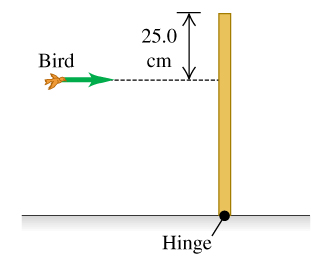# Problem: A 510.0 -g bird is flying horizontally at 2.30  m/s , not paying much attention, when it suddenly flies into a stationary vertical bar, hitting it 25.0 cm below the top (the figure ). The bar is uniform, 0.760 m long, has a mass of 1.90 kg , and is hinged at its base. The collision stuns the bird so that it just drops to the ground afterward (but soon recovers to fly happily away).a. What is the angular velocity of the bar just after it is hit by the bird?b. What is the angular velocity of the bar just as it reaches the ground?

###### FREE Expert Solution

In this problem, we'll use the concept of conservation of angular momentum.

$\overline{){{\mathbf{L}}}_{{\mathbf{i}}}{\mathbf{=}}{{\mathbf{L}}}_{{\mathbf{f}}}}$

a.

From the law of conservation of angular momentum:###### Problem Details

A 510.0 -g bird is flying horizontally at 2.30  m/s , not paying much attention, when it suddenly flies into a stationary vertical bar, hitting it 25.0 cm below the top (the figure). The bar is uniform, 0.760 m long, has a mass of 1.90 kg , and is hinged at its base. The collision stuns the bird so that it just drops to the ground afterward (but soon recovers to fly happily away).

a. What is the angular velocity of the bar just after it is hit by the bird?

b. What is the angular velocity of the bar just as it reaches the ground?